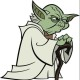# 创建一个空的 data.frame

``````df <- data.frame(Date=as.Date("01/01/2000", format="%m/%d/%Y"),
File="", User="", stringsAsFactors=FALSE)
df <- df[-1,]
``````770

``````df <- data.frame(Date=as.Date(character()),
File=character(),
User=character(),
stringsAsFactors=FALSE)
``````

``````df <- data.frame(Doubles=double(),
Ints=integer(),
Factors=factor(),
Logicals=logical(),
Characters=character(),
stringsAsFactors=FALSE)
str(df)
> str(df)
'data.frame':   0 obs. of  5 variables:
\$ Doubles   : num
\$ Ints      : int
\$ Factors   : Factor w/ 0 levels:
\$ Logicals  : logi
\$ Characters: chr
``````
N.B.:195

``````empty_df = df[FALSE,]
``````87

``````df = data.frame(matrix(vector(), 0, 3,
dimnames=list(c(), c("Date", "File", "User"))),
stringsAsFactors=F)
``````68

``````colClasses = c("Date", "character", "character")
col.names = c("Date", "File", "User")
colClasses = colClasses,
col.names = col.names)
``````

``````df <- read.csv(text="Date,File,User", colClasses = colClasses)
``````

(273)